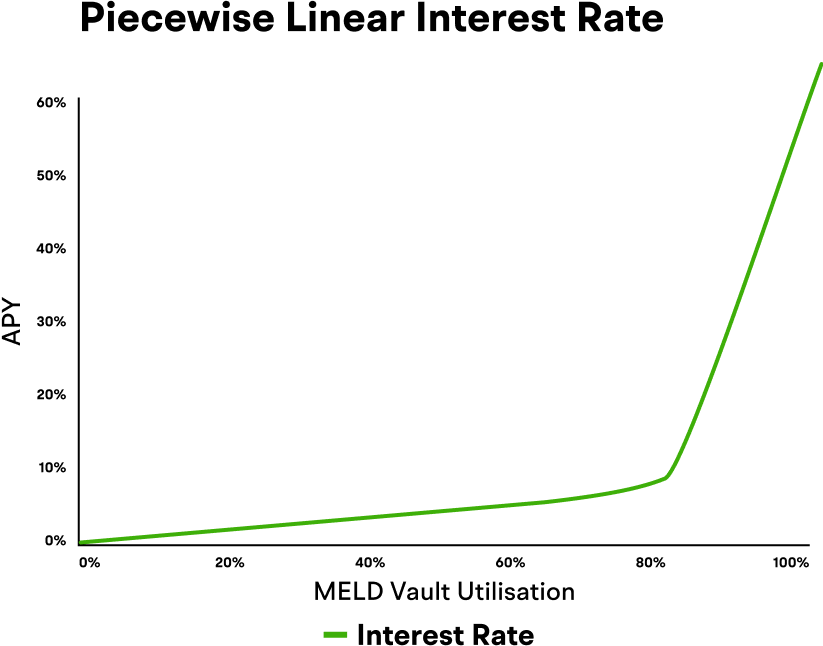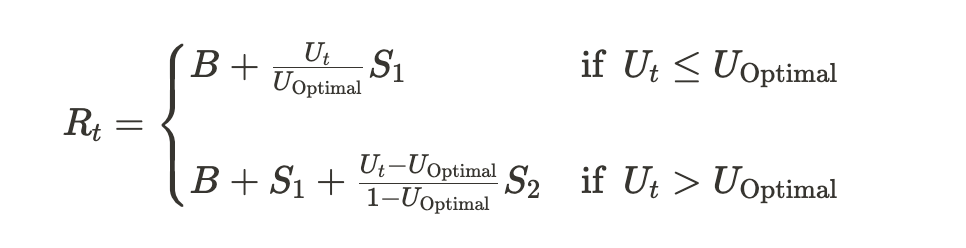# Yield Mechanism

Optimizing Returns Through Advanced DeFi Engineering

## Yield Mechanism

Both supply yield and loan interest are calculated and applied to collateral and debt respectively. This means that a user is able to supply an asset and the yield generated from this deposit is added to the total collateral the user is able to withdraw or borrow against. Likewise, the interest on the user’s loan is automatically added to the total outstanding debt. The interest rate is determined by the utilization rate of the pools, meaning that as people borrow more the interest rate goes up, and as people deposit more or repay their loans the interest rate goes back down.The interest rate is calculated using a set of protocol parameters, in particular a base rate, an initial slope, a secondary slope, and an optimal utilization rate. The interest rate is then calculated based on the current utilization rate as follows:The supply yield rate is determined by both the current interest rate and the current utilization rate, along with a parameter called the reserve factor, as follows:Our goal at MELD is to build a lending and borrowing protocol that leads to a user having a positive net interest rate. For example, a user deposits \$100 in AVAX for one year and the average annualized yield is 9% but the user has borrowed \$50 in USD and pay an average annualized interest rate of 4%. They will then have an annual net interest of 8%. They have borrowed but earned a yield along the way. This is the power of allowing users to be both suppliers of liquidity and borrowers at the same time.